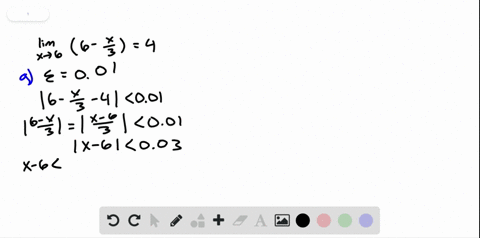Enroll in one of our FREE online STEM bootcamps. Join today and start acing your classes!View Bootcamps### Finding a $\delta$ for a Given $\varepsilon$ In E…

03:24University of California, Berkeley
Problem 39

# Finding a $\delta$ for a Given $\varepsilon$ In Exercises $39-44,$ find the limit $L .$ Then find $\delta$ such that $|f(x)-L|<\varepsilon$ whenever $0<|x-c|<\delta$ for (a $)$ $\varepsilon=0.01$ and \$(b) \varepsilon=0.005 .$$\lim _{x \rightarrow 4}(x+2)$$

## Discussion

You must be signed in to discuss.

## Video Transcript

Okay, so we have the limit as except for just two three x plus two is you go to eight. Let's write that as after a bout of after backs, which is three experts to minus elements, which is eight in less than we said, our no value 0.1 are after the absolute value. So we actually have to react. Is there a point there? One attacked her out of three. So let's see. Oh, actually, this should be a minus sign. Sorry about that. So three times up to a lot of extra minus is less than 0.1 note. Divide by three on both sides, and we get that girl is thus in explaining to is less then 0.1 divided by three, which is approximately point there. We'll go 33 and the contacts don't. So if we have Bill listen extra too dressed in Delta, which is equal to 0.33 then three time. That's the relative excellent, too. Indo 0.1 that is equal to three X minus six. Is that a no 0.1, which means that three x two minus eight. At that point, no one, which means that our function minus l is left in their appointed one